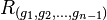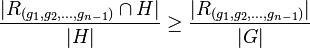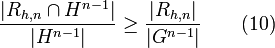# Difference between revisions of "Fraction of tuples satisfying groupy relation in subgroup is at least as much as in whole group"

View other such statements

## Statement

Suppose$G$ is a finite group. Suppose$R \subseteq G^n$ is a$n$-ary relation on$G$ with the property that if we consider a$n$-tuple$(g_1,g_2, \dots, g_i,\dots, g_n)$, and we fix all coordinates except the$i^{th}$ coordinate, the set of possibilities for$g_i$ forms a subgroup of$G$.

Suppose$H$ is a subgroup of$G$. Then, we have:$\! \frac{|H^n \cap R|}{|H^n|} \ge \frac{|R|}{|G^n|}$

In other words, the fraction of$n$-tuples from$H$ satisfying$R$ is at least as much as the fraction of$n$-tuples in$G$ satisfying$R$.

## Facts used

1. Index satisfies transfer inequality: This states that if$H,K \le G$, then$[H:H \cap K] \le [G:K]$. However, the form in which we use it is the conditional probability formulation, which states that:$\! \frac{|H \cap K|}{|H|} \ge \frac{|K|}{|G|}$

(Note: The letters$H,K$ have interchanged roles compared to the formulation of the result on its original page.)

## Proof

Given: Finite group$G$, groupy$n$-ary relation$R$ on$G$. Subgroup$H$ of$G$.

To prove:$\! \frac{|H^n \cap R|}{|H^n|} \ge \frac{|R|}{|G^n|}$

Proof: We prove the statement by induction on$n$. The proof for the base case$n = 1$ and the induction step are similar, but we give both proofs.

Proof of base case: In the base case, the relation has only one element, which means that it is just a subset$R$ of$G$. The groupiness now says that this subset is a subgroup.Then, fact (1) yields that:$\! \frac{|H|}{|H \cap R|} \le \frac{|G|}{|R|}$

Inverting both sides and flipping the inequality sign yields:$\! \frac{|H \cap R|}{|H|} \ge \frac{|R|}{|G|}$

Proof of induction step: Suppose we have shown the result for all$(n-1)$-ary relations. We now need to show it for the$n$-ary relation$R$.

Define$R_{(g_1,g_2,\dots,g_{n-1})}$ as the subset of$G$ comprising those values of$g_n$ such that$(g_1,g_2,\dots,g_{n-1},g_n) \in R$. Each such$R_{(g_1,g_2,\dots,g_{n-1})}$ is a subgroup, and:$\! |R| = \sum_{(g_1,g_2,\dots,g_{n-1}) \in G^{n-1}} |R_{(g_1,g_2,\dots,g_{n-1})}|\qquad (1)$

Also, we have:$\! |R \cap (G^{n-1} \times H)| = \sum_{(g_1,g_2,\dots,g_{n-1}) \in G^{n-1}} |R_{(g_1,g_2,\dots,g_{n-1})} \cap H| \qquad(2)$

Dividing both sides of (1) by$|G|$ and both sides of (2) by$|H|$, we get respectively:$\! \frac{|R|}{|G|} = \sum_{(g_1,g_2,\dots,g_{n-1}) \in G^{n-1}} \frac{|R_{(g_1,g_2,\dots,g_{n-1})}|}{|G|} \qquad (3)$$\! \frac{|R \cap (G^{n-1} \times H)|}{|H|} = \sum_{(g_1,g_2,\dots,g_{n-1}) \in G^{n-1}} \frac{|R_{(g_1,g_2,\dots,g_{n-1})} \cap H|}{|H|} \qquad(4)$

By fact (1), we have$[H:R_{(g_1,g_2,\dots,g_{n-1})} \cap H] \le [G:R_{(g_1,g_2,\dots,g_{n-1})}]$, yielding:$\! \frac{|R_{(g_1,g_2,\dots,g_{n-1})} \cap H|}{|H|} \ge \frac{|R_{(g_1,g_2,\dots,g_{n-1})}|}{|G|}$

Thus, each of the summands to (4) is bigger than the corresponding summand to (3), and thus we get:$\! \frac{|R \cap (G^{n-1} \times H)|}{|H|} \ge \frac{|R|}{|G|}$

Multiplying both denominators by$|G^{n-1}|$, we get:$\! \frac{|R \cap (G^{n-1} \times H)|}{|G^{n-1} \times H|} \ge \frac{|R|}{|G^n|} \qquad (5)$

For$g \in G$, define$R_{g,n}$ as the relation on$G^{n-1}$ induced by$R$ with the last coordinate$g$. Then:$\! |R \cap (G^{n-1} \times H)| = \sum_{h \in H} |R_{h,n}| \qquad (6)$

Finally, we have:$\! |R \cap H^n| = \sum_{h \in H} | R_{h,n} \cap H^{n-1}| \qquad (7)$

Dividing (6) and (7) by$|G^{n-1} \times H|$ and$|H^n|$ respectively, we get:$\! \frac{|R \cap (G^{n-1} \times H)|}{|G^{n-1} \times H|} = \frac{1}{|H|}\sum_{h \in H} \frac{|R_{h,n}|}{|G^{n-1}|}\qquad(8)$

and:$\! \frac{|R \cap H^n|}{|H^n|} = \frac{1}{|H|} \sum_{h \in H} \frac{|R_{h,n} \cap H^{n-1}|}{|H^{n-1}|}\qquad (9)$

Each$R_{h,n}$ is groupy. Thus, by induction, each$R_{h,n}$ satisfies:$\! \frac{|R_{h,n} \cap H^{n-1}|}{|H^{n-1}|}\ge \frac{|R_{h,n}|}{|G^{n-1}|} \qquad (10)$

Plugging (10) into the summations on the right sides of (8) and (9), we get:$\! \frac{|R \cap H^n|}{|H^n|} \ge \frac{|R \cap (G^{n-1} \times H)|}{|G^{n-1} \times H|} \qquad (11)$

Combining (5) and (11) yields the desired results.